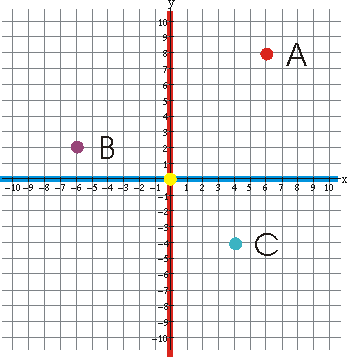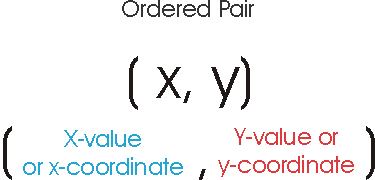The Coordinate Plane and its Parts

# The Coordinate PlaneA coordinate plane is formed by two lines (number lines). One is a horizontal number line going from left to right. This is called the x-axis. The other is a vertical number line going from the bottom to the top. This is called the y-axis. You can remember this if you remember "y goes high". The two number lines intersect at their zero points which forms the ordered pair (0,0) also called the point (0,0). This point is the only point with a fixed (unchanging - always the same) name and it is called the Origin. To name other points a single capital letter is used, examples are point A, point B,and point C.

# Ordered PairsThe first number in the ordered pair is the x-value or x-coordinate and the second is the y-value or y-coordinate. You can help yourself remember the order because it is alphabetical, x comes before y in the alphabet and it comes before y in an ordered pair. In an ordered pair the x-values and y-values can be positive, negative, or zero. Examples of orderd pairs are:
(-3, 4), (5,-8), (0,6), (-7,-7) and, (9,5).

This is called an ordered pair because the order of the numbers is important. If you put the numbers in the wrong spots the point or ordered pair is not always the same as you can see in the example below. The x-coordinate tells how far away from the y-axis a point is and the sign of the coordinate tells which side of the y-axis it is on (positive to the right, negative to the left). The y-coordinate tells how far from the x-axis the point is and the sign of the coordinate tells whether it is above or below the x-axis (positive is above and negative is below). You will see examples of this in the plotting points section later.

# Why is the order of the x-value and y-value important?The ordered pair (1,4) is not the same as the ordered pair (4,1). In the ordered pair (1,4) the 1 is the x-value and the 4 is the y-value. In the ordered pair (4,1) it is reversed, now the x-value is 4 not 1 like before and the y-value is 1 not 4 like before. the two points are not the same.

# Plotting Points in the Coordinate PlanePlotting points is easy, the point or ordered pair tells you what you need to do. Start by putting your pencil at the origin (the center of the graph, the yellow dot). The first value in the ordered pair is the x-value and there are two parts to it. The first part is the sign, it tells which direction to go, left if negative right if positive. The second part is the number it tells how many spaces to go. The same is true for the y-value, if it is positive go up as many spaces as the number says, if negative go down as many spaces as the number says. You are now at the spot where the point goes so put it there.

Look at the point A (6,8). To plot the point start at the origin. Move 6 spaces in the positive direction on the x axis and then 8 spaces up and then plot the point. For point B (-6,2) you start at the origin move 6 spaces in the negative direction along the x-axis and then go up 2 spaces and plot the point. For point C (4, -4) you start at the origin, move 4 spaces in the positive direction on the x-axis and then down 4 spaces and then plot the point.

# Quadrants of The Coordinate PlaneThe x-axis and y-axis divide the coordinate plane into four sections called quadrants. Sometimes they are labeled using Roman numerals I, II, III, and IV. Other times they are labeled Q1, Q2, Q3, and Q4. Either way is acceptable but it is easy to forget how to label them. Here is a way to help you remember: If you write the letter "C" in a coordinate plane so that the origin is inside the center of the "C" you will pass through the quadrants in the order they are supposed to be labeled. You start in the upper right-hand corner Q1 , then you pass through the upper left-hand corner Q2 , then you pass through the lower left-hand corner Q3 , and finally you end up in the bottom right-hand corner Q4 .

Look at the coordinate plane and notice that the values of the x-coordinates and y-coordinates are either positve or negative depending on the quadrant the point lies in. For instance the x-coordinate and y-coordinate are both positive for any point inside Q1 (quadrant 1). Is it possible for a point to be on the coordinate plane and not be in a quadrant? Yes. Have you ever seen a cat sitting on a fence teasing the dog in the yard below? The cat is not in any back yard, it is on the fence. Points are just like cats, they can be in a back yard (a quadrant) or they can be on the fence (on an axis). If a point is on the x-axis it has a y-coordinate of zero because it is not above or below the x-axis. If a point is on the y-axis it has an x-coordinate of zero because it is not to the left or right of the y-axis.